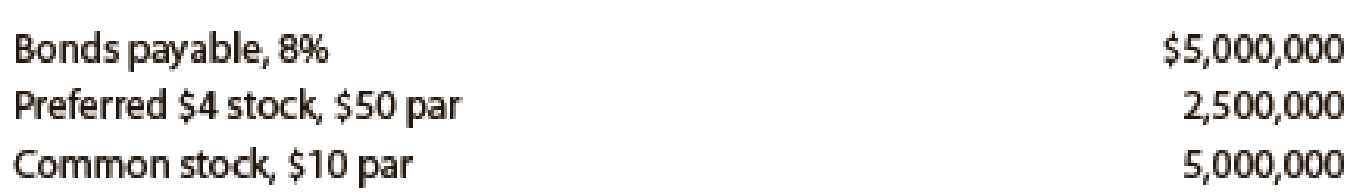Chapter 17, Problem 20E

Chapter
Section
Textbook Problem
1 views

# Five measures of solvency or profitabilityThe balance sheet for Garcon Inc. at the end of the current fiscal year indicated the following:Income before income tax was $3,000,000, and income taxes were$1,200,000 for the current year. Cash dividends paid on common stock during the current year totaled $1,200,000. The common stock was selling for$32 per share at the end of the year. Determine each of the following: (a) times interest earned ratio, (b) earnings per share on common stock, (c) price-earnings ratio, (d) dividends per share of common stock, and (e) dividend yield. Round ratios and percentages to one decimal place, except for per-share amounts.

To determine

Determine times interest earned ratio.

Explanation

Financial Ratios: Financial ratios are the metrics used to evaluate the liquidity, capabilities, profitability, and overall performance of a company.

Times interest earned ratio quantifies the number of times the earnings before interest and taxes can pay the interest expense. First, determine the sum of income before income tax and interest expense. Then, divide the sum by interest expense.

Formula:

Times-interest-earned ratio }=Income before income tax+Interest expenseInterest expense

Times interest earned ratio

Times-interest-earned ratio }

(b)

To determine

Determine earnings per share on the common stock.

(c)

To determine

Determine Price earnings ratio.

(d)

To determine

Determine Dividend per share of common stock.

(e)

To determine

Determine Dividend yield.

### Still sussing out bartleby?

Check out a sample textbook solution.

See a sample solution

#### The Solution to Your Study Problems

Bartleby provides explanations to thousands of textbook problems written by our experts, many with advanced degrees!

Get Started

#### Find more solutions based on key concepts